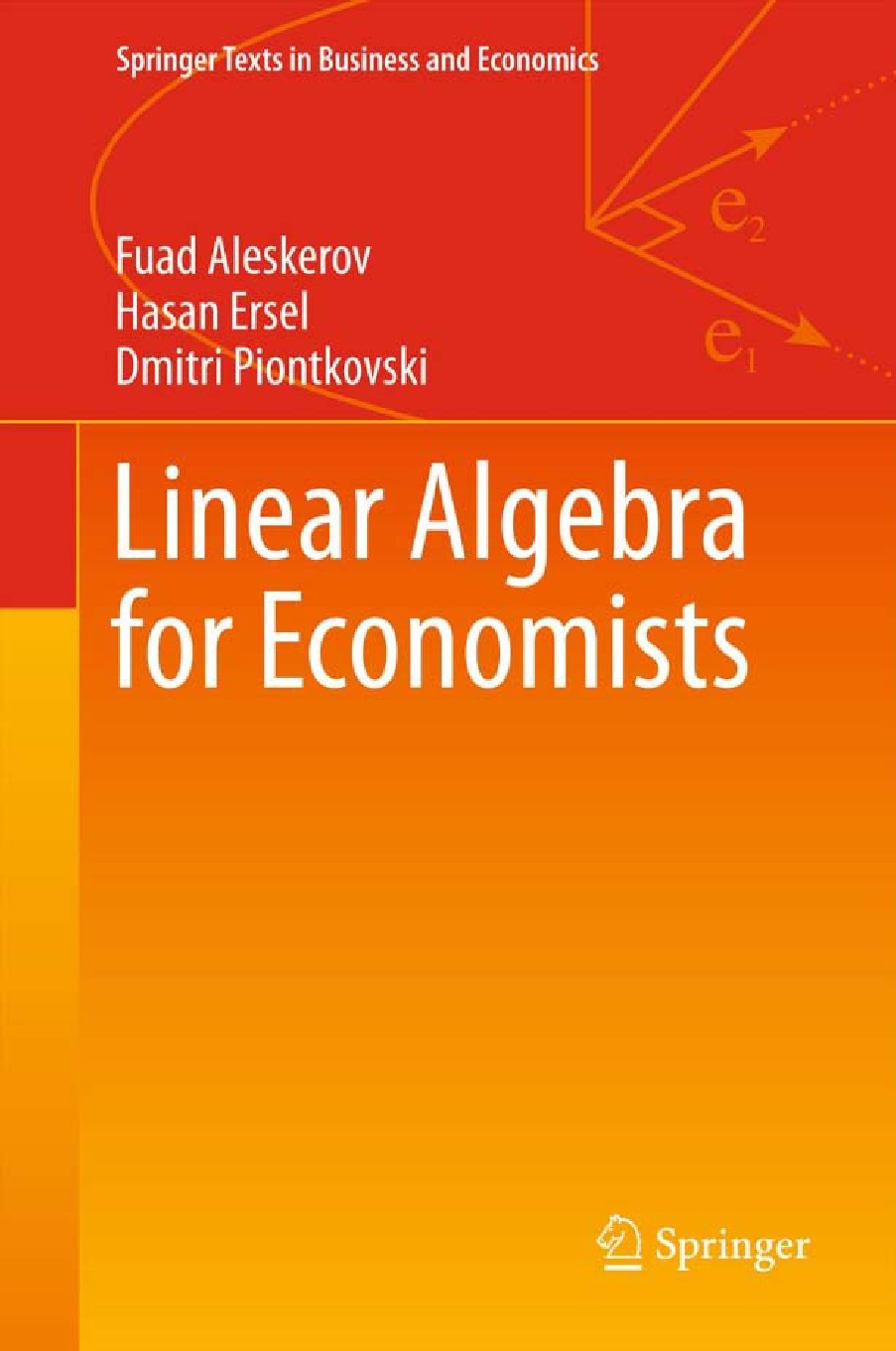• A
• A
• A
• ABC
• ABC
• ABC
• А
• А
• А
• А
• А
Regular version of the site

## Linear Algebra for Economists

Heidelberg; Dordrecht; L.; NY: Springer, 2011.

The main aim of the book is, naturally, to give students the fundamental notions and instruments in linear algebra. Linearity is the main assumption used in all fieldsof science. It gives a first approximation to any problem under study and is widely used in economics and other social sciences. One may wonder why we decided to write a book in linear algebra despite the fact that there are many excellent books such as [10, 11, 19, 27, 34]? Our reasons can be summarized as follows. First, we try to fit the course to the needs of the students in economics and the students in mathematics and informatics who would like to get more knowledge in economics. Second, we constructed all expositions in the book in such a way to help economics students to learn mathematics and the proof making in mathematics in a convenient and simple manner. Third, since the hours given to this course in economics departments are rather limited, we propose a slightly different way of teaching this course. Namely, we do not try to give all proofs of all theorems presented in the course. Those theorems which are not proved are illustrated via figures and examples, and we illustrated all notions appealing to geometric intuition. Those theorems which are proved are proved in a most accurate way as it is done for the students in mathematics. The main notions are always supported with economic examples. The book provides many exercises referring to pure mathematics and economics. The book consists of eleven chapters and five appendices. Chapter 1 contains the introduction to the course and basic concepts of vector and scalar. Chapter 2 introduces the notions of vectors and matrices, and discusses some core economic examples used throughout the book. Here we begin with the notion of scalar product of two vectors, define matrices and their ranks, consider elementary operations over matrices. Chapter 3 deals with special important matrices – square matrices and their determinants. Chapter 4 introduces inverse matrices. In Chap. 5 we analyze the systems of linear equations, give methods how to solve these systems. Chapter ends with the discussion of homogeneous equations. Chapter 6 discusses more general type of algebraic objects – linear spaces. Here the notion of linear independence of vectors is introduced, which is very important from economic point of view for it defines how diverse is the obtained information. We consider here the isomorphism of linear spaces and the notion of subspace. Chapter 7 deals with important case of linear spaces – the Euclidean ones. We consider the notion of orthogonal bases and use it to construct the idea of projection and, particularly, the least square method widely used in social sciences. In Chapter 8 we consider linear transformations, and all related notions such as an image and kernel of transformation. We also consider linear transformations with respect to different bases. Chapter 9 discusses eigenvalues and eigenvectors. Here we consider self-adjoint transformations, orthogonal transformations, quadratic forms and their geometric representation. Chapter 10 applies the concepts developed before to the linear production model in economics. To this end we use, particularly, Perron–Frobenius Theorem. Chapter 11 deals with the notion of convexity, and so-called separation theorems. We use this instrument to analyse the linear programming problem. We observe during the years of our teaching experience that induction argument creates some difficulties among students. So, we explain this argument in Appendix A. In Appendix B we discuss how to evaluate the determinants. In Appendix C we give a brief introduction to complex numbers, which are important for better understanding the eigenvalues of linear operators. In Appendix D we consider the notion of the pseudoinverse, or generalized inverse matrix, widely used in different economic applications. Each chapter endswith the number of problemswhich allowbetter understanding the issues considered. In Appendix E the answers and hints to solutions to the problems from previous chapters and appendices are given.